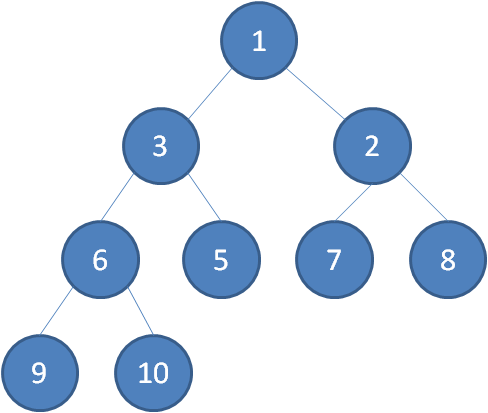# 数据结构之二叉树与二叉堆

## 数据结构之二叉树与二叉堆

A、每个结点都有一个指向其第一个孩子的指针
B、每个结点都有一个指向其第一个右兄弟的指针1.最小堆（父结点的值，小于左右结点）2.最大堆（父结点的值，大于左右结点）a.插入操作（首先在末尾添加结点 再通过上浮操作 将结点放在合适的地方）b.删除操作（比如要删除 堆顶元素 为了保持完全二叉树的结构 用最后一个结点补到堆顶位置（删除结点处）再进行下沉操作 ）c.构建二叉堆

• 左孩子=parent*2+1;
• 右孩子=parent*2+2;
• parent=（孩子-1）/2 //左右孩子都是

``````public class HeapOperator {

public static void upAdjust(int[] array) {

int childIndex=array.length-1;
int parentIndex=(childIndex-1);

//temp保持插入的叶子节点值，用于最后赋值
int temp=array[childIndex];

//childIndex不是堆顶 并且 子节点的值 小于父结点
//进行上浮操作
while(childIndex>0&&temp<array[parentIndex])
{

//无需真正交换 将被替换的节点 赋给上浮的子节点就行
array[childIndex]=array[parentIndex];
childIndex=parentIndex;
parentIndex=(parentIndex-1)/2; //变小

}

//若是上浮成功 对上浮来的子节点赋值
array[childIndex]=temp;
}
/** * 下沉调整 * @param array 待调整的堆 * @param parentIndex 要下沉的父节点 * @param parentIndex 堆的有效大小 */
public static void downAdjust(int[] array, int parentIndex, int length) {

//保持父节点的值用于最后的赋值
int temp=array[parentIndex];
int childIndex=2*parentIndex+1;

while(childIndex<length) //存在子节点
{

//存在右孩子 并且右孩子的值小于左孩子 定位到右孩子
if(childIndex+1 <length&&array[childIndex-1]<array[childIndex])
{

childIndex++;
}
//如果temp 小于任何一个结点的值直接跳出

if(temp <array[childIndex])

{

break;
}

//无需进行真正交换
array[parentIndex]=array[childIndex];
parentIndex=childIndex;
childIndex=2*childIndex+1;   //变大了

}
array[parentIndex]=temp;
}
/** * 构建堆 * @param array 待调整的堆 */
public static void buildHeap(int[] array) {

//从底层的最后一个非叶子节点进行下沉操作
//这样效率偏低
// for (int i=(array.length-2)/2;i>=0;i--)
// {

// }

//这样理论更快点
for(int i=0;i<=(array.length-2)/2;i++)
{

}
}
public static void main(String[] args) {

//二叉堆创建
//非叶子结点 大值下沉
long startTime=System.currentTimeMillis();//记录开始时间
int[] array =new int[]{
7,1,3,10,5,2,8,9,60};
buildHeap(array);
System.out.println(Arrays.toString(array));

long endTime=System.currentTimeMillis();//记录结束时间
float excTime=(float)(endTime-startTime)/1000;
System.out.println("执行时间："+excTime+"s");

//节点添加
array=new int[]{
1,3,2,6,5,7,8,9,10,0};
System.out.println(Arrays.toString(array));
}
}
``````

• 1.在末尾添加一个结点 通过优先级 进行上浮操作
• 2.完成堆顶节点（队列） 删除节点（栈顶与最后一个节点交换）时，通过下沉操作 会根据优先级选举出新的堆顶
• 3.遍历删除 会将最小堆变成最大堆
``````public class HeapSort {

public static void downAdjust(int[] array, int parentIndex, int length) {

//保持父节点的值用于最后的赋值
int temp=array[parentIndex];
int childIndex=2*parentIndex+1;

while(childIndex<length) //存在子节点
{

//存在右孩子 并且右孩子的值小于左孩子 定位到右孩子
if(childIndex+1 <length&&array[childIndex-1]<array[childIndex])
{

childIndex++;
}
//如果temp 小于任何一个结点的值直接跳出

if(temp <array[childIndex])

{

break;
}

//无需进行真正交换
array[parentIndex]=array[childIndex];
parentIndex=childIndex;
childIndex=2*childIndex+1;   //变大了

}
array[parentIndex]=temp;
}

public static void heapSort(int [] array)
{

//进行下沉操作
for(int i=0;i<=(array.length-2)/2;i++)
{

}
System.out.println(Arrays.toString(array));

//循环删除堆顶元素

for(int i=array.length-1;i>0;i--)
{

//最后一个元素与第一个元素交换
int temp=array[i];
array[i]=array;
array=temp;
//下沉调整最大堆

}

}

public static void main(String[] args) {

int[] arr=new int[]{
1,3,2,6,5,7,8,9,10,0};
//先变成最小堆
//再通过删除栈顶变成了 最大堆
heapSort(arr);
System.out.println(Arrays.toString(arr));

}
}
``````